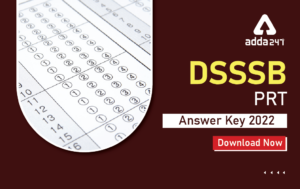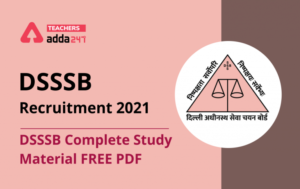Home   »   Reasoning Questions For DSSSB/HSSC Exam |...

# Reasoning Questions For DSSSB/HSSC Exam | 28th September 2019The reasoning is the most scoring section in any competitive exam. It also develops or nourishes our critical thinking skills as compared to other subjects. In DSSSBKVSAPS Exam as a student to score well in this part, you have to start your preparation now because there is negative marking as well. The more you have prepared, the better you score.

Directions (1-2): In each of the following questions, select the related word/letters/number from the given alternatives.

Q1. BEHI: JMPQ ∷ KNQR:?
(a) SUXY
(b) RUXY
(c) RVXY
(d) RUYX

Q2. 625: 25 ∷ 225:?
(a) 13
(b) 14
(c) 15
(d) 16

Directions (3-5): In each of the following questions, select the one which is different from the other three responses.

Q3.
(a) Wood
(b) Cork
(c) Stone
(d) Paper

Q4.
(a) Rain
(b) Cloud
(c) Mist
(d) Fog

Q5.
(a) 92 – 99
(b) 48 – 54
(c) 58 – 64
(d) 89 – 95

Q6. Arrange the following words according to English Dictionary.
(A) PREMONITION
(B) PRELUDE
(C) PREMICE
(D) PRELIMINARY
(a) DBAEC
(b) BDCEA
(c) DBCEA
(d) BDACE

Q7. In the following questions, choose the correct alternative from the given ones that will complete the series.
1, 6, 13, 22, ?, 46
(a) 32
(b) 33
(c) 40
(d) 43

Q8. A and B are the young ones of C. If C is the mother of B, but A is not the daughter of C, then what is the relationship between C and A?
(a) Nephew and Aunty
(b) Brother and Sister
(c) Mother and Son
(d) Niece and Aunty

Q9. Which interchange of signs or numbers will make the following equation correct?
(7 + 2) × 3 × 4 – 1 = 20
(a) 2 and 3
(b) × and –
(c) 7 and 3
(d) + and ×

Q10. Select the correct combination of mathematical signs to replace * signs and to balance the given equation.
6 * 15 * 10 * 3 * 12
(a) ÷ + = ×
(b) + ÷ × =
(c) × ÷ + =
(d) + – = ÷

Solutions

S1. Ans.(b)

Sol.S2. Ans.(c)S3. Ans.(c)
Sol. Stone is different from the other three. Cork and Paper are made up with wood and wood pulp.

S4. Ans.(a)
Sol. Rain is different from the other three.

S5. Ans.(a)
Sol.
99 – 92 = 7
54 – 48 = 6
64 – 58 = 6
95 – 89 = 6

S6. Ans.(c)
Sol. Arrange of Words as per dictionary:
(D) PRELIMINARY

(B) PRELUDE

(C) PREMICE

(A) PREMONITION

S7. Ans.(b)
Sol.S8. Ans.(c)
Sol. C is the mother of A and B. A is son of C.

S9. Ans.(d)
Sol. (7 + 2) × 3 × 4 – 1 = 20
⇒ (7 × 2) + 3 + 4 – 1 = 20
⇒ 14 + 3 + 4 – 1 = 20

S10. Ans.(c)
Sol. 6 * 15 * 10 * 3 * 12
⇒ 6 × 15 ÷ 10 + 3 = 12
⇒ 9 + 3 = 12

You may also like to read :

Sharing is caring!

Thank You, Your details have been submitted we will get back to you.
•DSSSB PRT Answer Key 2022 Released @dsss...
•DSSSB Complete Study Material PDF Free
•DSSSB TGT PGT Result 2022 Out: Check TGT...
•DSSSB Eligibility 2022 Check Post-Wise E...
•DSSSB TGT PGT Result 2022 Out: Check TGT...
•DSSSB Eligibility 2022 Check Post-Wise E...